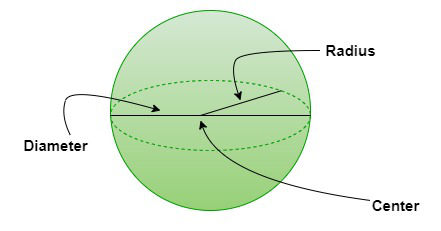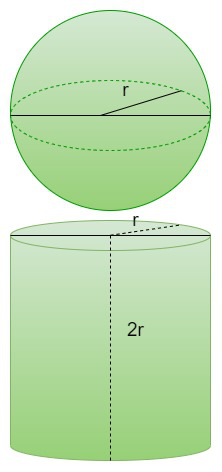Open in App
Not now

# Surface Area of a Sphere

• Last Updated : 25 Aug, 2022

The surface area of a sphere is the area occupied by the curved surface of that sphere. In geometry, a sphere is a three-dimensional solid figure that is round in shape, with every point on its surface equidistant from its center. For example, the globe, ball, and so on. The distance between any point on the surface of a sphere and its center is called the “radius of a sphere.” A sphere is a three-dimensional figure that is defined in three dimensions, i.e., the x-axis, the y-axis, and the z-axis, whereas a circle is a two-dimensional shape that is defined in a plane.

## What is the Surface Area of a Sphere?

The surface area of a sphere is the region covered by the outer surface in the 3-dimensional space. It can be said that a sphere is the 3-dimensional form of a circle. The surface area of a sphere formula is given in terms of pi (π) and radius.

Surface Area of a Sphere = 4πr2 square units

Where, r = radius of the sphere.## Surface Area of a Sphere Formula

The surface area of a sphere is calculated using the radius of the sphere. If the surface area of the sphere is “S” and the radius is “r”, then the formula for surface area of a sphere is:

Surface area of a sphere = 4πr2

If the diameter of the sphere is given instead of the radius, the formula will become:

Surface area of a sphere = πd2

## Derivation of Surface Area of a Sphere

The area occupied by the surface of a sphere in space is the surface area of a sphere. We know that a sphere is round in shape, so to calculate its surface area, we can connect it to a curved shape, such as a cylinder. A cylinder is a three-dimensional figure that has a curved surface with two flat surfaces on either side. Let’s consider that the radius of the sphere and the radius of a cylinder is the same. So the sphere can perfectly fit into a cylinder. Therefore, the height of the sphere is equal to the height of a cylinder, i.e., the diameter of a sphere. This fact was proved by the mathematician Archimedes that the surface area of a sphere of radius “r” is equal to the lateral surface area of a cylinder of radius “r”. Therefore,The Surface area of a sphere = The Lateral surface area of a cylinder

We know that,

The lateral surface area of a cylinder = 2πrh,

Where r is the radius of the cylinder and h is its height.

We have assumed that the sphere perfectly fits into the cylinder. So, the height of the cylinder is equal to the diameter of the sphere.

Height of the cylinder (h) = Diameter of the sphere (d) = 2r (where r is the radius)

Therefore,

The Surface area of a sphere = The Lateral surface area of a cylinder = 2πrh

Surface area of the sphere = 2πr × (2r) = 4πr2

Hence,

The surface area of the sphere = 4πr2 square units

Where r = radius of the sphere

## How to Find the Surface Area of a Sphere

The surface area of a sphere is simply the area occupied by its surface. Let’s consider an example to see how to determine the surface area of a sphere using its formula.

Example: Find the surface area of a sphere of radius 7 cm.

Step 1: Note the radius of the given sphere. Here, the radius of the sphere is 47 cm.

Step 2: We know that the surface area of a sphere = 4πr2. So, substitute the value of the given radius in the equation = 4 × (3.14) × (7)2 = 616 cm2.

Step 3: Hence, the surface area of the sphere is 616 square cm.

## Curved Surface Area of a Sphere

The sphere has only one curved surface. Therefore, the curved surface area of the sphere is equal to the total surface area of the sphere, which is equal to the surface area of the sphere in general. Therefore, it is safe to say that,

Curved Surface Area of a Sphere = 4πr2

## Solved Examples on Surface Area of Sphere

Example 1: Calculate the total surface area of a sphere with a radius of 15 cm. (Take π = 3.14)

Solution:

Given, the radius of the sphere = 15 cm

We know that the total surface area of a sphere = 4 π r2 square units

= 4 × (3.14) × (15)2

= 2826 cm2

Hence, the total surface area of the sphere is 2826 cm2.

Example 2: Calculate the diameter of a sphere whose surface area is 616 square inches. (Take π = 22/7)

Solution:

Given, the curved surface area of the sphere = 616 sq. in

We know,

The total surface area of a sphere = 4 π r2 square units

⇒ 4 π r2 = 616

⇒ 4 × (22/7) × r2 = 616

⇒ r2 = (616 × 7)/(4 × 22) = 49

⇒ r = √49 = 7 in

We know, diameter = 2 × radius = 2 × 7 = 14 inches

Hence, the diameter of the sphere is 14 inches.

Example 3: Find the cost required to paint a ball that is in the shape of a sphere with a radius of 10 cm. The painting cost of the ball is ₨ 4 per square cm. (Take π = 3.14)

Solution:

Given, the radius of the ball = 10 cm

We know that,

The surface area of a sphere = 4 π r2 square units

= 4 × (3.14) × (10)2

= 1256 square cm

Hence, the total cost to paint the ball = 4 × 1256 = ₨ 5024/-

Example 4: Find the surface area of a sphere whose diameter is 21 cm. (Take π = 22/7)

Solution:

Given, the diameter of a sphere is 21 cm

We know,

⇒ 21 = 2 × r ⇒ r = 10.5 cm

Now, the surface area of a sphere = 4 π r2 square units

= 4 × (22/7) × (10.5)

= 1386 sq. cm

Hence, the total surface area of the sphere = 1386 sq. cm

Example 5: Find the ratio between the surface areas of two spheres whose radii are in the ratio of 4:3. (Take π = 22/7)

Solution:

Given, the ratio between the radii of two spheres = 4:3

We know that,

The surface area of a sphere = 4 π r2

From the equation, we can say that the surface area of a sphere is directly proportional to the square of its radius.

⇒ A1/A2 = (r1)2/(r2)2

⇒ A1/A2 = (4)2/(3)2 = 16/9

Therefore, the ratio between the total surface areas of the given two spheres is 16:9.

Example 6: Find the ratio between the radii of two spheres when their surface areas are in the ratio of 25:121. (Take π = 22/7)

Solution:

Given, the ratio between the total surface areas of two spheres = 25:121

We know that,

The total surface area of a sphere = 4 π r2

From the equation, we can say that the surface area of a sphere is directly proportional to the square of its radius.

⇒ r1/r2 = √A1/√A2

⇒ r1/r2 = √25/√121 = 5/11

Therefore, the ratio between the radii of the given two spheres is 5:11.

## FAQs on Surface Area of Sphere

Question 1: How to find the surface area of a sphere?

The surface area of a sphere is given by the formula,

Surface area = 4πr2

Where r is the radius of the sphere.

Question 2: What is the surface area of a hemisphere?

The surface area of a hemisphere is given by the sum of half of the sphere’s surface area and the base area, that is,

S.A. = 2πr2 + πr2

The surface area of a hemisphere = 3πr2

Question 3: What is the lateral surface area of a sphere?